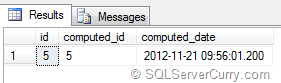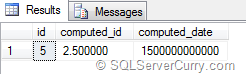### Computed Columns in SQL Server

Computed columns are derived columns that are bound to values of other columns. However the datatype of these computed columns depends on the nature of the end result. They may be bound to columns of either the same datatype or they could be bound to columns of different datatypes.

Consider the following set of data

declare @t table(id int, computed_id as id, computed_date as dateadd(day,id,getdate()))
insert into @t (id)
select 5
select * from @t

The result isAs you can see, the datatype of computed_id will be same as that of the ID as ID is directly used in the computed column definition. However the datatype of the column computed_date will be datetime because the expression dateadd(day,id,getdate()) will do an implicit conversion to datetime datatype as getdate() is used in the definition.

Let us consider another set of data

declare @t table(id int, computed_id as id/2.0, computed_date as id*300000000000)
insert into @t (id)
select 5
select * from @t

The output isAs you can see, the datatype of computed_id will be of decimal type because of the expression id/2.0 which results to decimal number. The datatype of the column computed_numberf will be BIGINT because the expression id*300000000000 will do an implicit conversion to the BIGINT datatype as the result won't fit into a INT datatype

So the datatpye of computed column differs based on the expression and if you want to update the value returned by a computed column to another table, you need to make sure that the datatypes match each other.

Keep these points in mind while using Computed columns in SQL Server